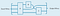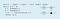# New powers: From bit to qubit

By Will Zeng: Software & Applications @Rigetti Computing

Read this first: The Quantum Software Challenge.

This is the second of a series of posts on the software stack for quantum computing. This post is a quick reminder of what’s new about quantum computing and why this world is different.

If quantum computing is totally new to you, then the Economist has an accessible article on recent developments with an appropriate wink & nudge title: A little bit, better.

# New powers

Quantum computers open up new powers of computation that are completely inaccessible to regular computers. Here’s a pair of simple examples:

• Say you have 100 boxes and you know that one element is hidden in them. In the worst case, how many boxes do you need to look in to open the box with the element? It seems obvious that the answer should be 100. A quantum computer only needs to look in 10 boxes. More generally, given N boxes, a quantum computer only needs to look in square root of N boxes. Over a million boxes this amounts to a 1000x speedup. This is achieved with Grover’s algorithm.
• Suppose you wanted to exactly simulate a small molecule — say 300 electron orbitals — to extract out some properties like its ground state energy. Maybe that molecule is important for photosynthesis and could help us build cheaper and more efficient solar panels, or maybe it could help us design catalysts to pull harmful carbon out of the atmosphere. A traditional computer that is the size of the observable universe could not begin to solve this problem. A quantum computer with hundreds of qubits, could, though exactly how long it will take depends on the quality of its software.

You can read more about these and other applications in our quantum software post.

# From bit to qubit

People usually talk about quantum computing protocols using the quantum circuit framework. A quantum circuit is the quantum analog to a boolean circuit and describes a sequence of gates performed on one or several registers of qubits.A simple quantum circuit. The wires represent a qubit or register of qubits and the boxes are different operations (gates) performed on them. The crossing wires represent a SWAP gate. We usually read circuits from left to right.

We can get a window into the power of quantum computing even at the level of individual bits. A classical bit has a state value of 0 or 1, and we can represent these values as state vectors |0> and |1>.

A probabilistic classical bit can then be represented by a sum of these state vectors: p|0> + q|1>, where p and q are real numbers in the unit interval that represent the probability of finding that bit in either state, e.g. the bit has a probability p of being found in the |0> state. Clearly we always have p + q = 1 and the state ½|0>+½|1> represents a uniformly random bit. Of course when we measure the state it is actually only in state 0 or 1 after measurement.

A quantum bit or qubit has a similar structure. It has two output states |0> and |1> and a generic qubit state is represented as a sum of vectors a|0> + b|1>, except now the weights a and b are complex numbers so that

|a|^2 + |b|^2 = 1.

The probability of the qubit being in state |0> is given by |a|^2 and similarly |b|^2 gives the probability of observing outcome |1>.

Consider our uniformly random classical bit. There’s only one state that gives uniformly output statistics, while in the quantum case there are several, where d = \sqrt{2}:

1/d |0> + 1/d |1>
1/d |0> -1/d |1>
1/d |0> + i/d |1>
1/d |0> -i/d |1>

Mathematically we represent qubit states as vectors whose elements are the coefficients are a and b. Quantum gates are then matrices, which have behavior that differs from Boolean gates. One important example is the Hadamard gate, H:

The Hadamard gate produces superpositions. For example if we send in a qubit that is set to |0> we obtain one of the uniform superpositions given above:

A similar argument shows that the Hadamard gate operating on a qubit in the the |1> state also produces a uniform superposition, but a different one.

All these different states produce the same measurement statistics as the uniformly random bit. This hints that a qubit has many more exotic states than usual bits. There’s a beautiful geometric representation of these extra states in the Bloch sphere: the states of a classical bit can be represented as a vector on the unit line, and the extra states for a single qubit can be represented as vectors on a unit sphere. The usual output states of |0> and |1> then reside on the north and south poles. The classical bit can only be measured along one axis, but the qubit has many options and these different kinds of measurements cause many other different exotic behaviors. For more, see Aaronson’s book or Nielsen and Chuang’s quantum computing bible.

Further, the state space of qubits scales astoundingly quickly compared to traditional bits. This scaling comes from the fact that every n-qubit state vector has 2^n complex weights, so in some sense n qubits can store 2^n classical bits because (unlike the 2^n real weights in a probabilistic classical vector) the qubit is actually in one of those 2^n states. Mathematically, qubit states and quantum gates compose via the tensor product. Importantly though, due to subtleties worth reading about, we can only ever extract n classical bits of information from n qubits, despite these new states.

This makes quantum algorithms apt for studying global properties of functions and data. One can construct some large superposition which encodes many possibilities, and then return the answer to a question about some global property without ever returning the whole superposition.

High-level languages turn these algorithms into quantum circuits for execution on hardware. We’ll talk more about this later.Some code for a quantum protocol (written in Quipper) that generates an output circuit.

Now for the layers. They’re self-contained, so feel free to jump around.

Written by